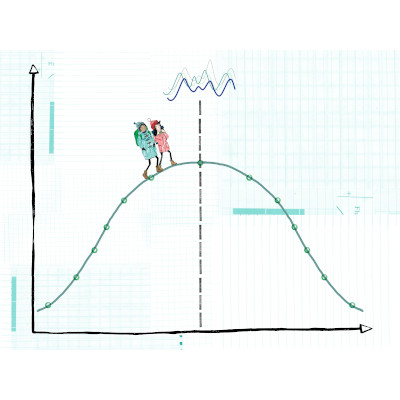GitHub

# Training Course Details## Introduction to Bayesian Inference

### Course Level: Foundation

The capturing and quantification of uncertainty is a very important aspect of model-fitting and parameter inference. Bayesian inference represents a fully-probabilistic approach to parameter inference, allowing a practitioner to quantify their uncertainties through probability densities. However, fitting models in a Bayesian framework can be an involved and complicated affair, often necessitating the use of Markov chain Monte Carlo (MCMC) algorithms and their programmatic implementation.

## Introduction to Bayesian Inference

 Start Date: Price: Venue Details: Time: Duration:

## Course Details

• Course Outline
• Learning Outcomes
• Materials
• Prior Knowledge
• Bayesian inference: Motivation of the Bayesian philosophy and an introduction to Bayes’ Theorem.
• Markov chain Monte Carlo (MCMC) methods: An overview of MCMC methods and the problems they seek to overcome.
• Posterior predictive simulation: Capturing uncertainty about the predictions of a model.

By the end of this course participants will…

• Understand the merits of the Bayesian workflow and the importance of uncertainty quantification.
• Have developed an intuitive understanding of prior beliefs, the likelihood function, and the posterior distribution.
• Be able to articulate the difficulties of fitting models in a Bayesian framework.
• Understand how MCMC algorithms work and how they can be used to alleviate difficulties in model-fitting.
•••••Prior to attending this course, participants should be familiar with basic concepts of probability and statistics, including common probability distributions and regression methods.

## Attendee Feedback

• “High standard of teaching made challenging theory seem simple!”